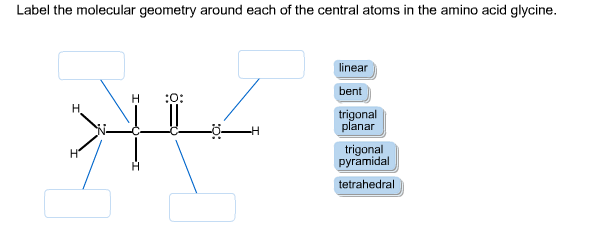# Label the molecular shape around each of the central atoms in the amino acid glycine.Label the molecular geometry around each of the central atoms in the amino acid glycine.

General guidance

Concepts and reason
The concept used here is that the phenomenon of hybridization is use in valence bond theory. In hybridization, mixing of atomic orbitals takes place to as a result of suitable pairing of electrons to form chemical bonds. Orbitals involved in these bonds are known as hybridized orbitals. With the help of hybrid orbitals, molecular geometry (bond angles) and atomic bonding properties can be determined. Practically, we can find bond angles with the help of various spectroscopic and diffraction methods but theoretically, it can also be determined by the use of Valence shell electron-pair repulsion (VSEPR) theory. In this method, we first draw the Lewis structure of the given compound and then count the number of band pairs and lone pairs present in the structure.

Fundamentals

VSEPR theory is applied by seeing the electron pairs surrounded on that particular atom. The theory for VSEPR is that the atom adopts an arrangement so as to minimizes the repulsion occur due to its surrounding electron.
Lewis structure is a diagram which represents atoms of a molecule and the lone pairs present in the molecule.
Bond angles and geometry of a molecule can be determined with the help of this table:

Also Read :   298, 209, 129, 58, -4..whats the next number in the series?

Step-by-step

Step 1 of 4

Hybridization on nitrogen is . So, geometry around nitrogen on position (1) is trigonal pyramidal.

Nitrogen on position (1) has 1 lone pair and 3 bond pair of electron, so their sum is 4. According to table 1, it belongs to trigonal pyramidal.

Sum of bond pair and lone pair is 4 in this case. It also belongs to tetrahedral geometry but the presence of lone pair shifts this geometry to trigonal pyramidal to attain stability.

Check Carbon at position (2) for geometry.

Step 2 of 4

Hybridization of Carbon at position (2) is . So, geometry aroundCarbon at position (2) is tetrahedral.

Carbon at position (2) has 4 bond pair and zero lone pair of electrons. According to Table 1, it has hybridization and have tetrahedral geometry.

Carbon at position (2) has only 4 bond pair of electrons. In tetrahedral geometry,it has maximum stability.

Check Carbon at position (3) for geometry.

Step 3 of 4

Hybridization of Carbon at position (3) is and has trigonal planer geometry.

Also Read :   cos’è la profondità d’animo?

Carbon at position (3) has 3 bond pair and zero lone pair of electrons. According to table 1, hybridization is and has trigonal planer geometry.

In bond pair counting, only sigma bond is considered. So, in case of double bonds only 1 bond pair is counted.

Check oxygen at position (4) for geometry

Step 4 of 4

Hybridization of Oxygen at position (4) is and has bent geometry.

Labelled molecular structure of Glycine is:

Oxygen at position (4) has 2 bond pair and 2 lone pair of electrons. According to table 1, hybridization is and has bent geometry.

Oxygen here attain bent geometry to attain stability as there is lone pair – lone pair repulsion which make the structure unstable.

Labelled molecular structure of Glycine is: